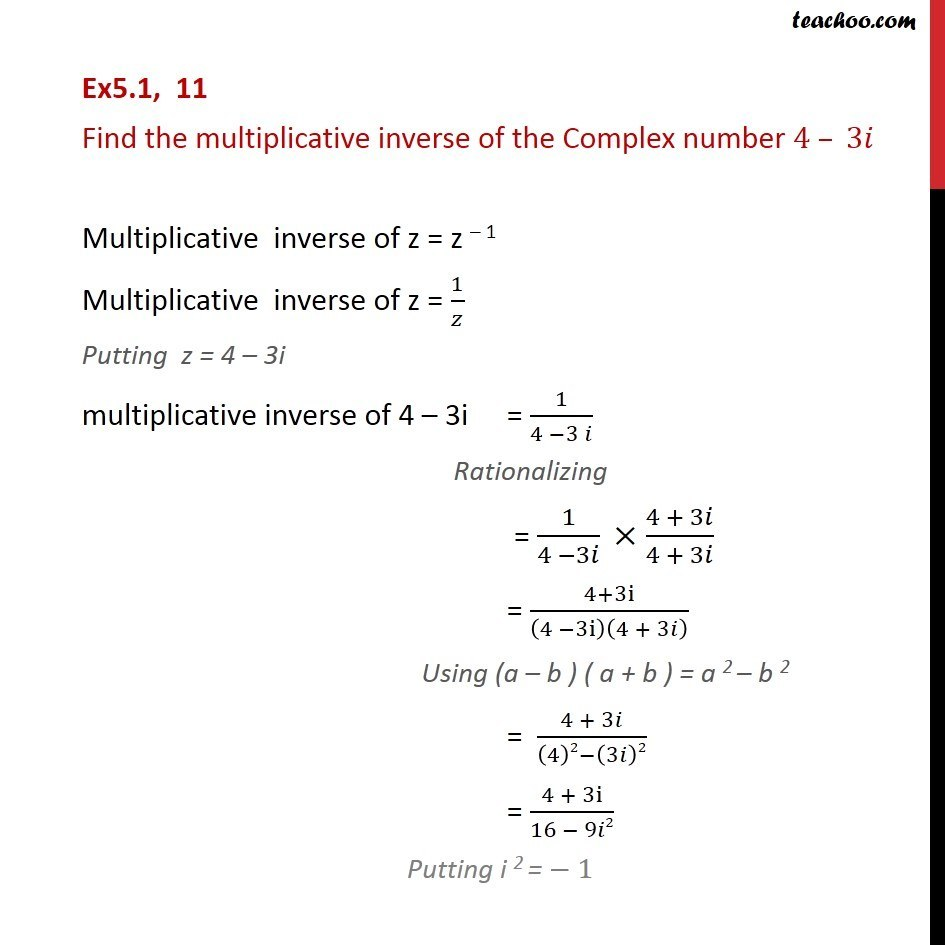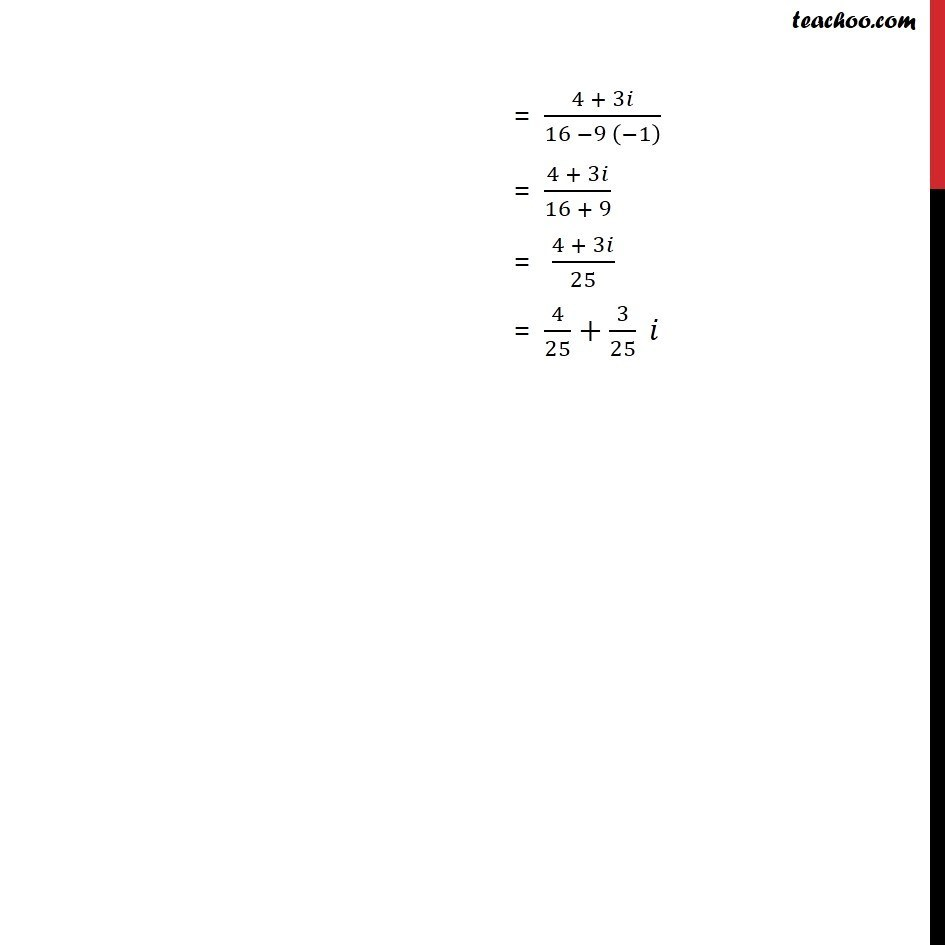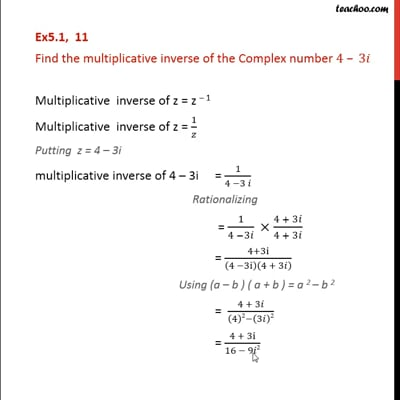Ex 5.1

Chapter 5 Class 11 Complex Numbers
Serial order wiseThis video is only available for Teachoo black users

Solve all your doubts with Teachoo Black (new monthly pack available now!)

### Transcript

Ex5.1, 11 Find the multiplicative inverse of the Complex number 4 – 3𝑖 Multiplicative inverse of z = z – 1 Multiplicative inverse of z = 1/𝑧 Putting z = 4 – 3i multiplicative inverse of 4 – 3i = 1/(4 −3 𝑖) Rationalizing = 1/(4 −3𝑖) ×(4 + 3𝑖)/(4 + 3𝑖) = (4+3i)/(4 −3i)(4 + 3𝑖) Using (a – b ) ( a + b ) = a 2 – b 2 = (4 + 3𝑖)/((4)2−(3𝑖)2) = (4 + 3i)/(16 − 9𝑖2) Putting i 2 = − 1 = (4 + 3𝑖)/(16 −9 (−1) ) = (4 + 3𝑖)/(16 + 9) = (4 + 3𝑖)/25 = 4/25+3/25 𝑖